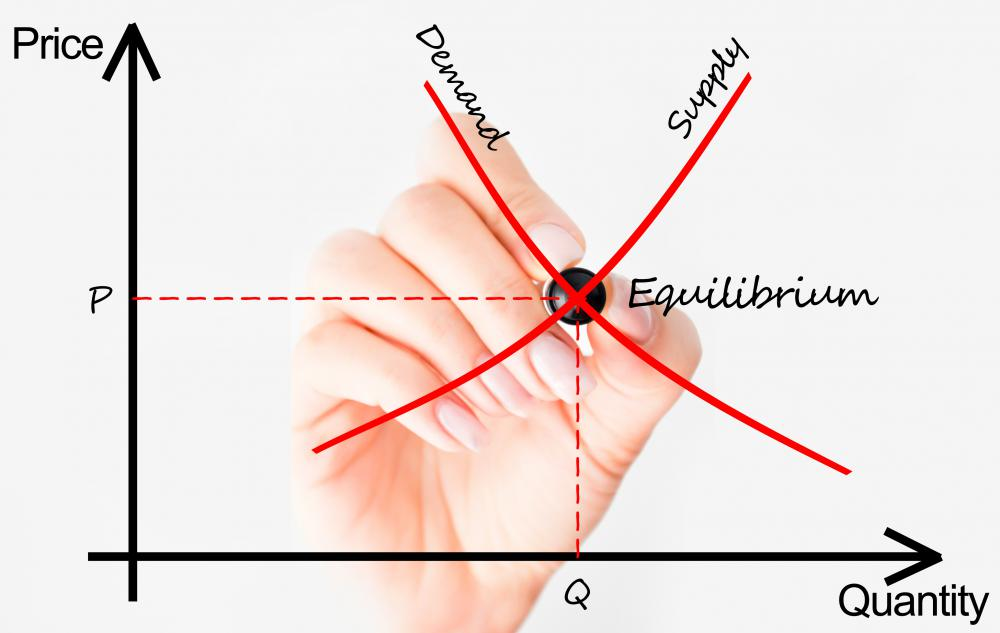# What Is the Relationship between Average Cost and Marginal Cost?

C. Daw

The basic average cost and marginal cost of a company are very different concepts, but they work together and fluctuate up and down according to how the other cost grows or declines. Average cost can be described as the ratio of the total cost to the total number of goods sold. It is equal to the total cost of the goods sold divided by the number of items sold. It has a very strong relationship with the supply and demand curves. Average cost can also be described as the sum of the average variable costs and average fixed costs. On the other hand, marginal cost is the cost that has incurred due to an additional unit or product. Average cost and marginal cost are inter-related because when the marginal cost goes up, or down, the average cost will fluctuate as well.Supply and demand influence average price; changes in output influence marginal cost of a product..

Average cost is different from the actual price because it depends upon the overall relationship between supply and demand. In some situations, price can be lower than the average cost, depending upon the marginal cost. Marginal cost is the variable cost of producing an additional unit. When it is greater than the average cost, then the marginal cost pulls the average upwards. If it is lower than the average cost, then the marginal cost pulls the average cost downward. These are the relationships between these two entities. When average cost and marginal cost are of same value, then the average cost remains constant, without any change.Average cost has a strong relationship with supply and demand curves.

If the average cost grows with the increase in the outputs, then the company has an increasing cost. The expenses would grow as the costs of management and the coordination of the employees gets tough and complex. On the other hand, if the average costs decrease with the passage of time, while the output increases, the costs would decrease. This could be done by adding more specialists into the employees’ category and implementing customized plans. The supply curve is very important considering the relationship between the average cost and marginal cost. The supply curve of an organization can be regarded as that portion of the marginal cost curve that lies above the average variable cost.

Average cost and marginal cost are both related to each other. Marginal cost is ever changing parameter, since it can fluctuate with the changes in the output. It is the ratio of the change in total cost to the change in output. The average total cost decreases in the start but then increases as a general behavior. It depends upon the average variable costs and the average fixed costs since it is the sum of them. If the average variable cost rises then the average total cost may go up, but only when the average variable cost is greater than the average fixed cost. The average cost will also not increase even if the average variable cost increases abruptly, which may be due to the rapid decrease of the average fixed cost.

## You might also Likejmiller2

More simply put, the average cost is often more than the marginal cost, because the average cost includes the initial start up costs, such as the purchase of a car factory, tools and parts, while the marginal cost might only include the cost of additional car materials and the labor required to build one more car. The marginal cost and average cost could be equal for simple items such as friendship bracelets where the only cost is thread and crafting time.